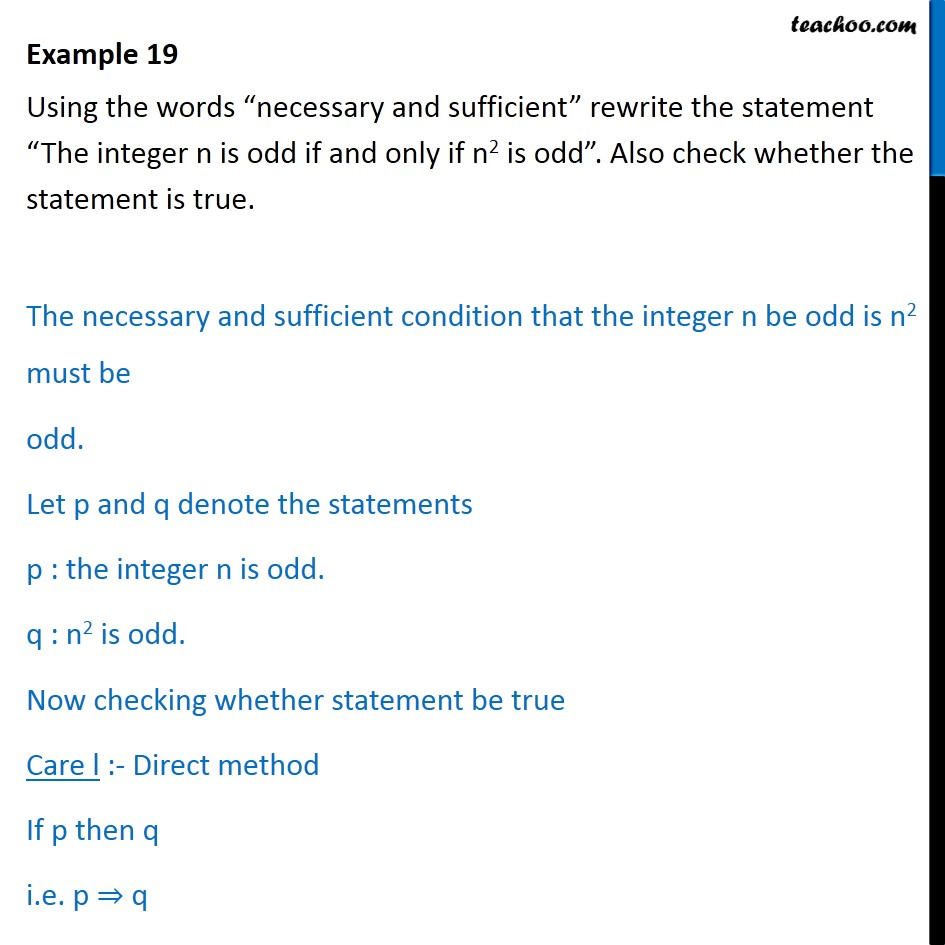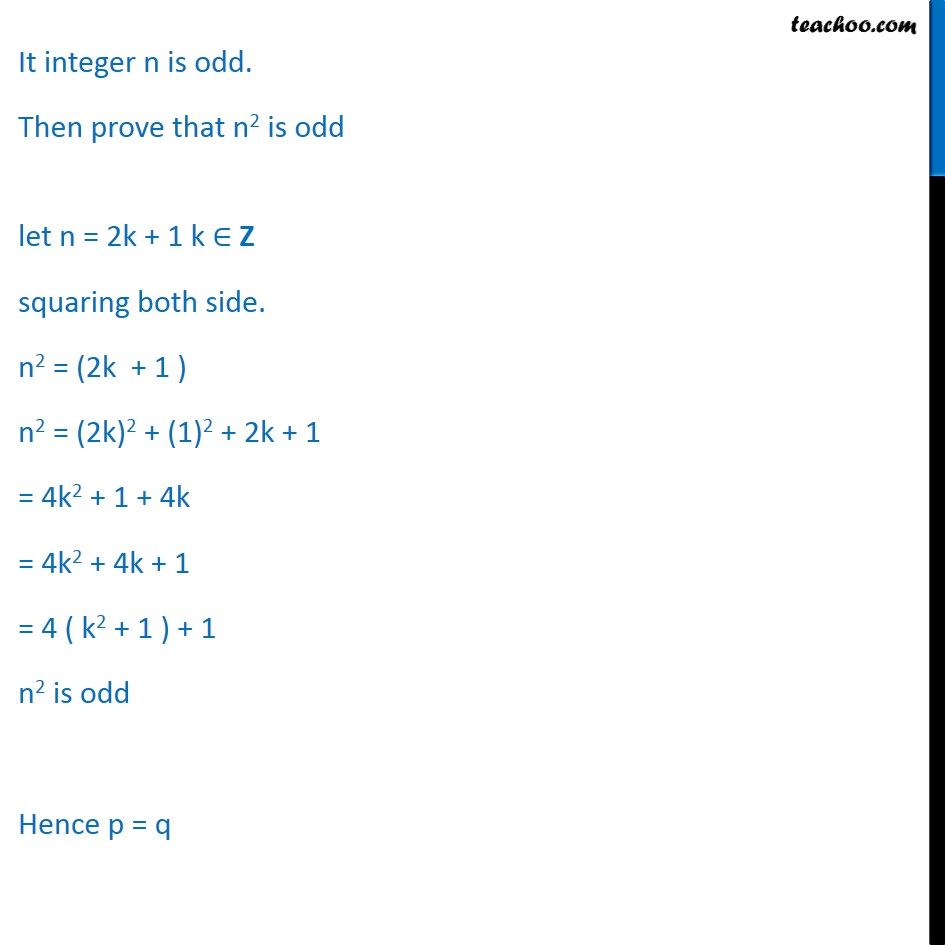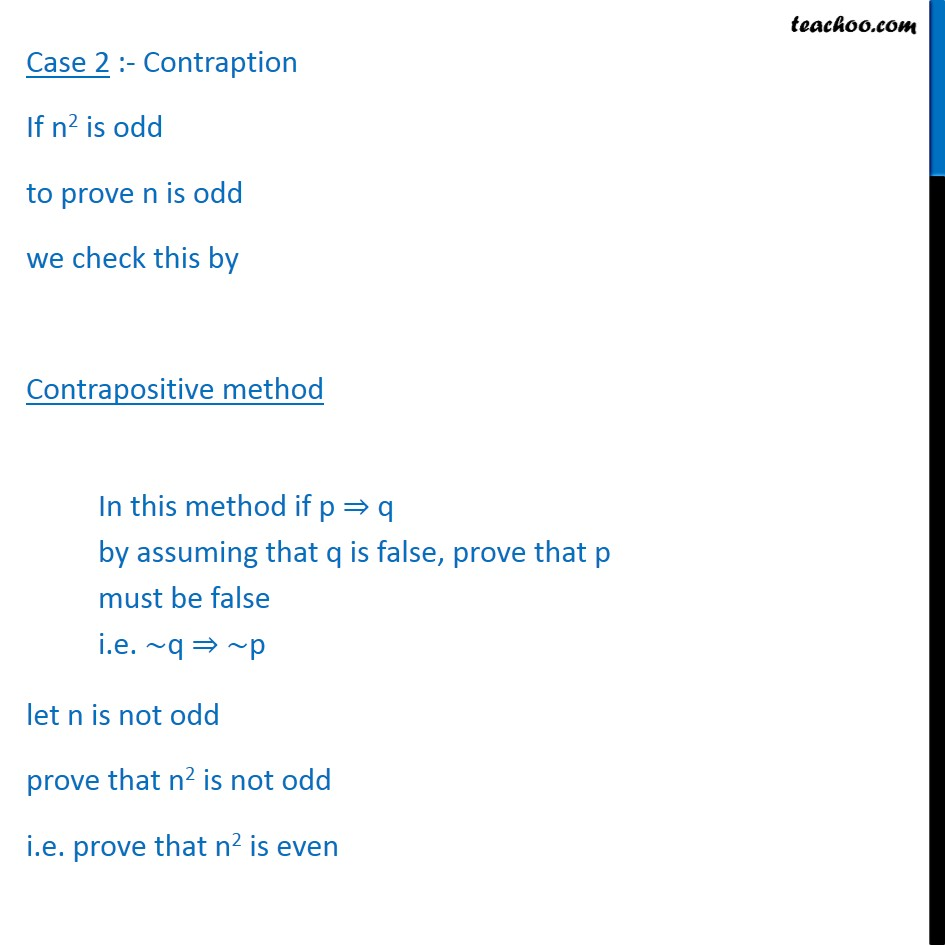Examples

Mathematical Reasoning
Serial order wiseLearn in your speed, with individual attention - Teachoo Maths 1-on-1 Class

### Transcript

Example 19 Using the words necessary and sufficient rewrite the statement The integer n is odd if and only if n2 is odd . Also check whether the statement is true. The necessary and sufficient condition that the integer n be odd is n2 must be odd. Let p and q denote the statements p : the integer n is odd. q : n2 is odd. Now checking whether statement be true Care l :- Direct method If p then q i.e. p q It integer n is odd. Then prove that n2 is odd let n = 2k + 1 k Z squaring both side. n2 = (2k + 1 ) n2 = (2k)2 + (1)2 + 2k + 1 = 4k2 + 1 + 4k = 4k2 + 4k + 1 = 4 ( k2 + 1 ) + 1 n2 is odd Hence p = q Case 2 :- Contraption If n2 is odd to prove n is odd we check this by Contrapositive method let n is not odd prove that n2 is not odd i.e. prove that n2 is even i.e. prove that n2 is even Now let n is not odd i.e. n is even i.e. n = 2k Squaring both side n2 = (2k)2 n2 = 4k2 This show n2 is even Hence n2 is not odd